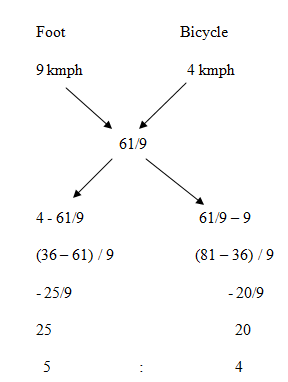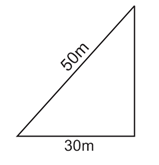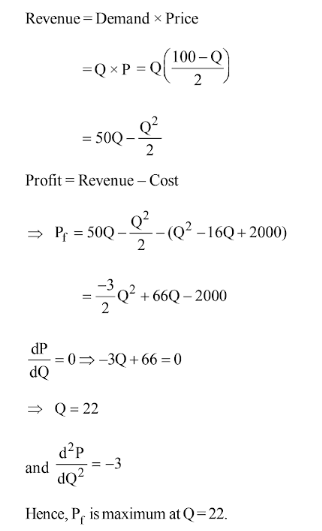# SSC CGL EXAMS 2018 | Quantitative Aptitude Practice Questions (Day-18)

Dear Aspirants, Here we have given the Important SSC Exam 2018 Practice Test Papers. Candidates those who are preparing for SSC 2018 can practice these questions to get more confidence to Crack SSC 2018 Examination.

[WpProQuiz 4731]

1) Which of the following least value should be added to 1440 to make it divisible by 3, 5, and 11?

a) 45

b) 12

c) 120

d) 34

2) P, Q and R can complete a work in 10, 12 and 15 days respectively. All three of them starts together but after 2 days P leaves the job and Q left the job 3 days before the work was complete.  R completes the remaining work alone. In how many days was the total work complete?

a) 5 days

b) 10 days

c) 7 days

d) 4 days

3) One of the diagonal of a rhombus is 70% of the other diagonal. What is the ratio of area of rhombus to the square of the length of the larger diagonal?

a) 3: 19

b) 3: 10

c) 7: 20

d) 7: 10

4) A gun is fried at a distance of 0.125 km from Amir. He hears the sound after 5 seconds. The speed (in kmph) at which sound travels is

a) 100 kmph

b) 125kmph

c)75 kmph

d) 90 kmph

5) A Boy travelled a distance of 61 km in 9 hours. He travelled partly on foot at the rate of 9 kmph and partly on bicycle at the rate of 4 kmph. The distance travelled in km on foot is:

a) 25 km

b) 16 km

c) 45 km

d) 54 km

6) The horizontal distance of a kite from the boy flying it is 30m and 50 m of cord is out from the roll. If the wind moves the kite horizontally at the rate of 5 km/h directly away from the boy, how fast is the cord being released?

1) 3 km/h

2) 4 km/h

3) 5 km/h

4) 6 km/h

7) If the ratio of the roots of the equation x2 –2ax + b = 0 is equal to that of the roots x2 –2x + d = 0, then

a) a2b = c2d

b) a2c = b2d

c) a2d = c2b

d) d2b = c2a

8) The sum of the numbers from 1 to 100, which are not divisible by 3 and 5 is.

a) 2946

b) 2732

c) 2632

d) 2317

9) As of now, what is the profit maximizing output?

a) 22

b) 21.5

c) 20

d) 19

10) If the Government imposes the 25% corporate profit tax, then what will be the profit maximizing output?

a) 16.5

b) 16.125

c) 15

d) 22

LCM of 3, 5 and 11 = 165

Now on dividing 2266 by 165, we get

1440 = 165 * 8 + 120

Thus, the least value which should be added to 2266 to make it divisible by 5, 7 and 11 = 165 – 120 = 45

The whole work = x days

Time taken by P = 10 days

Q = 12 days

R = 15 days

LCM of 10, 12, 15 = 60

Total work = 60 Unit

P can complete 60/10 = 6 units/ day

Q = 5 units/ day

R = 4 units/ day

P’s (2x) + Q’s (x – 3) + R (x) = 60

6 (2) + 5 (x – 3) + 4 (x) = 60

12 + 5x – 15 + 4x = 60

9x – 3 = 60

x = 63/9

x = 7 days

So, the whole work will be completed in 7 days.

Let the larger Diagonal of rhombus = a

Other diagonal = 70a/100 = 7a/10

Area of rhombus : Square of larger diagonal

½ Larger diagonal * smaller Diagonal : (larger Diagonal)2

½ * a * 7a/10 : a2

7a2 : 20a2

7: 20

Distance traveled by Bullet = 0.125 km = 125 m

Time taken = 5 sec

Speed = Distance / time = 125/ 5 = 25 mps * (18/5)

= 5*18 = 90 Kmph

Total Distance = 61 km

Total time = 9 hours

Relative Speed = 61/9 kmph

Speed of the boy travelled on Foot = 4 kmph

Speed of the boy travelled on bicycle = 9 kmph

On Alligation,Time ratio of On foot and bicycle = 4x : 5x

Time taken of the boy travelled on foot = = 4 hours

Speed of the boy to travel on foot = 9 kmph

Total distance = Speed * time = 4 * 9 = 45 km

Since, speed of wind = 5 km/hIt is passing horizontally for the will be less than the speed of the wind and will be in the same ratio

If the ratio of roots is n : 1

Then, the product of roots = n2 : 1 = b : d

Sum of the roots = a : c will be in ratio = n : 1

b/d = a2/c2

a2d = c2b

Sum of number 1 to 100 = 100/2 (1 + 100)

= 50 * 101 = 5050

Number which are divisible by 3 from 1 to 100

5, 10, 15, ………., 100

Sn = 20/2 (5 + 100)

= 10 * 105 = 1050

Number which is divisible by 3 and 5 must be divisible by 3 5 = 15 that are 15, 30,………, 90

Sn = 6/2 (15/90)

= 3 * 105 = 315

Sum of number which is divisible by 3 and 5 are = 1050 + 1683 – 315 = 2418

Sum of number which are not divisible by 3 and 5 = 5050 – 2418 = 2632

(i) The demand for a product (Q) is related to the price (P) of the products as follows Q =100 – 2P

(ii) The cost (C) of manufacturing the product is related to the quantity produced in the following manner C = (Q2 – 16Q + 2000)

(iii) As of now the corporate profit tax rate is zero. But the government of India thinking of imposing 25% tax on the profit of the company.Even after imposing tax on profit the profit maximizing output will still be 22.

### *******************

#### SSC CGL EXAMS 2018 |Quantitative Aptitude Practice Questions (Day-15)